# Math in Focus Grade 4 Chapter 9 Practice 3 Answer Key Turns and Right Angles

Practice the problems of Math in Focus Grade 4 Workbook Answer Key Chapter 9 Practice 3 Turns and Right Angles to score better marks in the exam.

## Math in Focus Grade 4 Chapter 9 Practice 3 Answer Key Turns and Right Angles

Find the measure of each angle.

Question 1.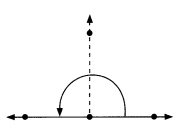A $$\frac{1}{2}$$-turn is ____.
180°.

Explanation:
Here, a $$\frac{1}{2}$$-turn is 180°.

Question 2.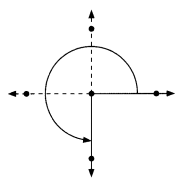A $$\frac{3}{4}$$-turn is ____.
270°.

Explanation:
Here, a $$\frac{3}{4}$$-turn is 270°.

Fill in the blanks.

Question 3.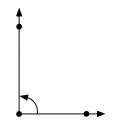A ___ -turn is 90°
$$\frac{1}{4}$$.

Explanation:
Here, a $$\frac{1}{4}$$-turn is 90°.

Question 4.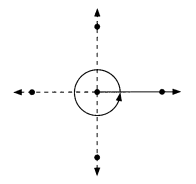A ___ -turn is 360°
1 -turn.

Explanation:
Here, a 1 -turn is 360°.

Look at the three pairs of angle strips shown.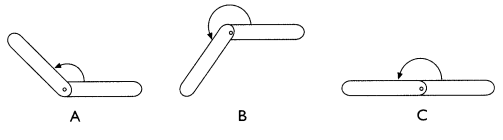Which pair of angle strips shows

Question 5.
$$\frac{1}{2}$$ turn? ___________
∠c.

Explanation:
In the above images, ∠c is a $$\frac{1}{2}$$ turn which is 180°.

Question 6.
a straight angle? ____
∠c.

Explanation:
In the above images, ∠c is a $$\frac{1}{2}$$ turn which is 180°.

Question 7.
a turn between $$\frac{1}{2}$$-turn and $$\frac{3}{4}$$-turn? ____
225°.

Explanation:
a turn between $$\frac{1}{2}$$-turn and $$\frac{3}{4}$$-turn is 225°.

Look at the three pairs of angle strips shown.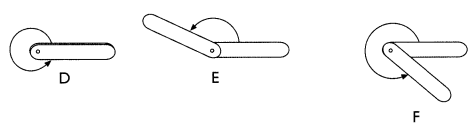Which pair of angle strips shows

Question 8.
360°? _________
1 turn.

Explanation:
In the above images, ∠D is a 1 turn which is 360°.

Question 9.
an angle between 180° and 360°? ___________
$$\frac{3}{4}$$.

Explanation:
In the above images, ∠F is a $$\frac{3}{4}$$ turn which is 270°.

Complete.

Question 10.
180° makes up ___ of a full turn.
180° makes up half of a full turn.

Explanation:
Here, 180° makes up half of a full turn. As 360° makes a full turn.

Question 11.
Three right angles make up a __ -turn
Three right angles make up a $$\frac{3}{4}$$ -turn.
Here, three right angles make up a $$\frac{3}{4}$$ -turn which is 270°.
105° is between a $$\frac{1}{4}$$-turn and a $$\frac{1}{2}$$-turn.
105° angle lies between a $$\frac{1}{4}$$-turn and a $$\frac{1}{2}$$-turn.